Publicité

# Unit1 pg math model

Program Coordinator National Board of Accreditation at PVPIT, Bavdhan Pune à PVPIT
3 May 2013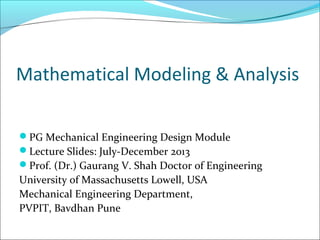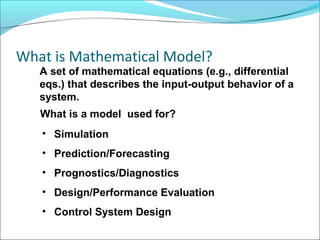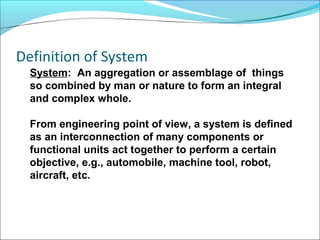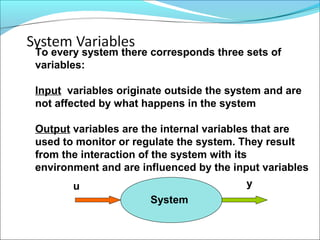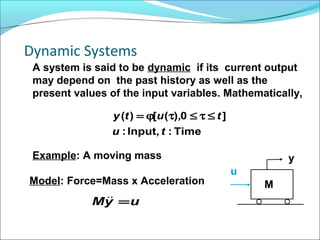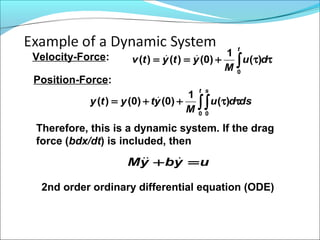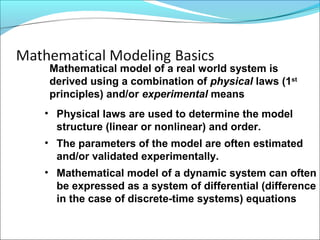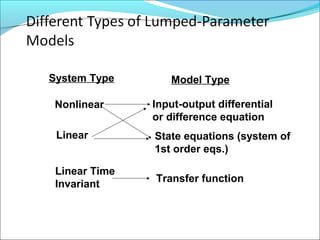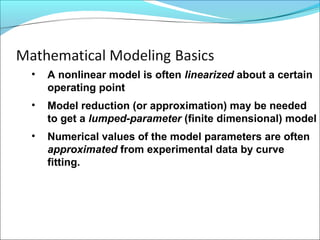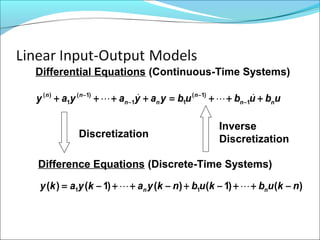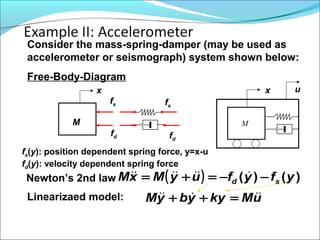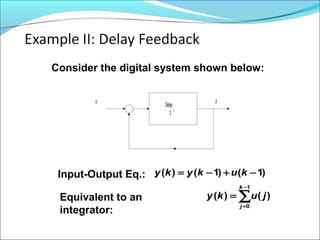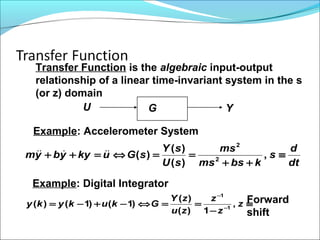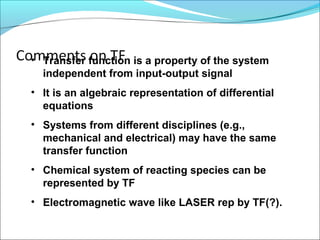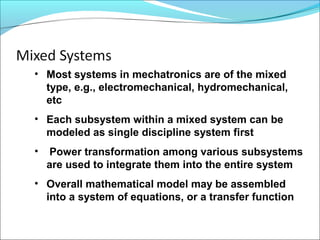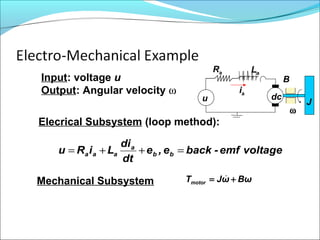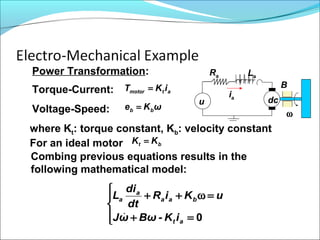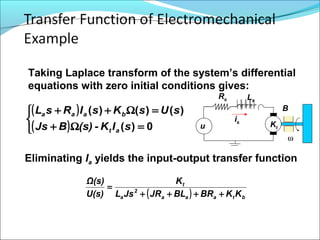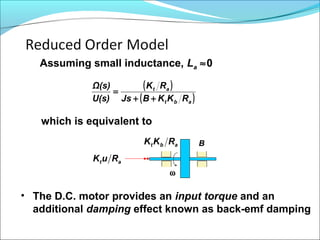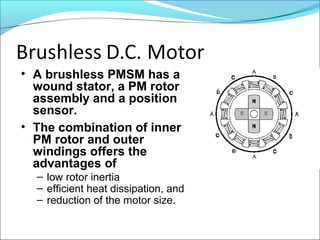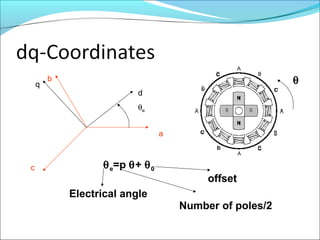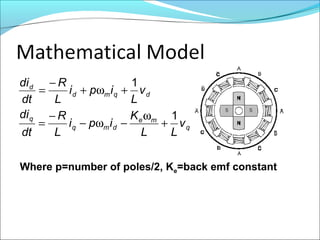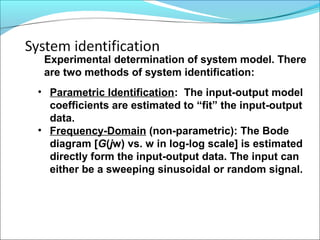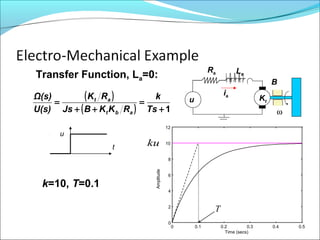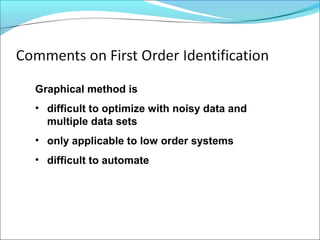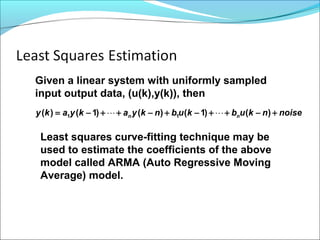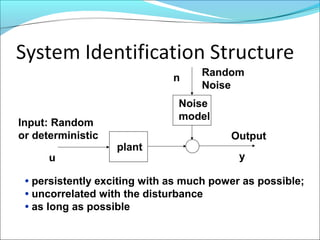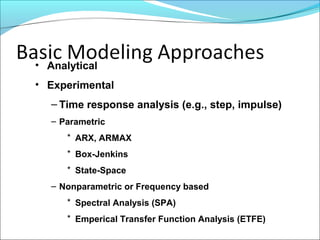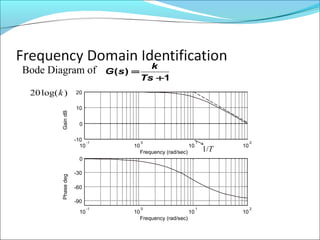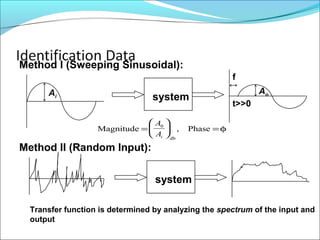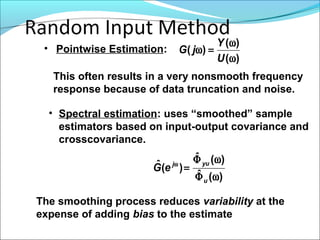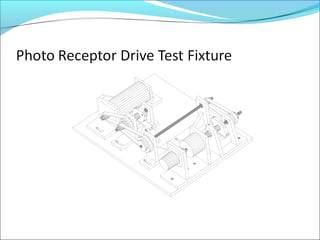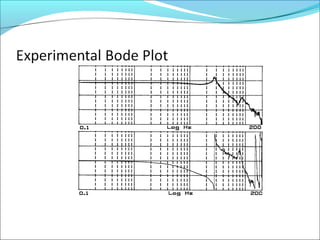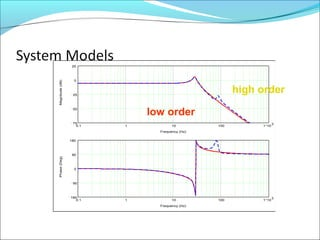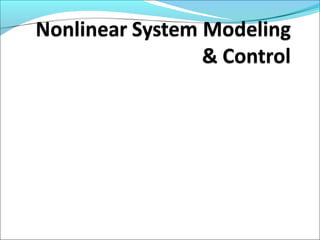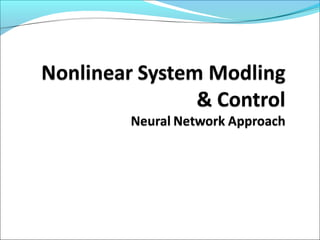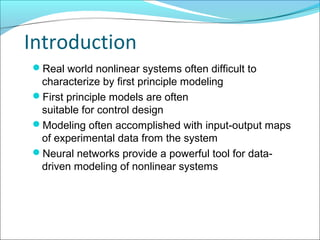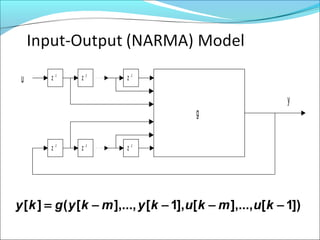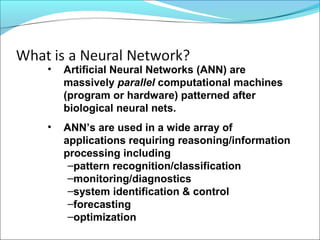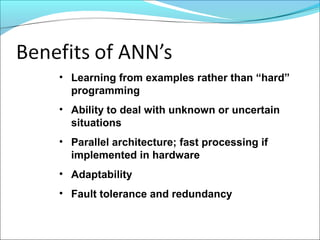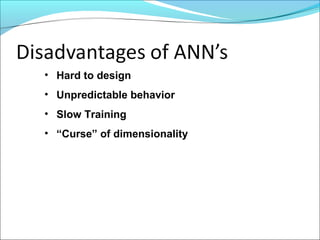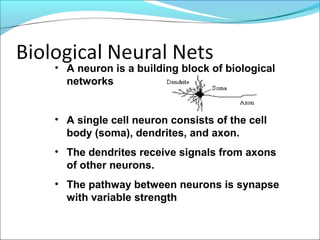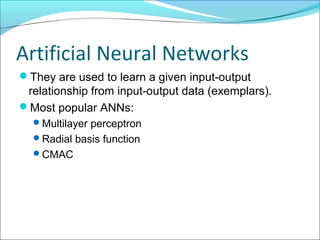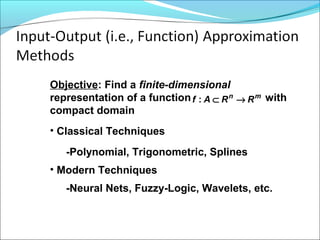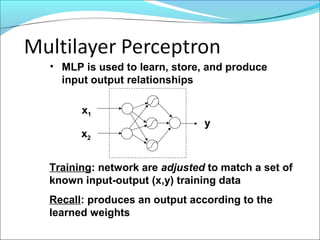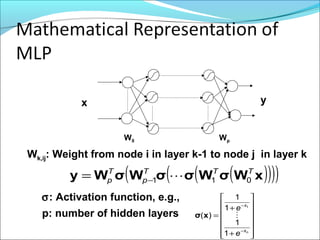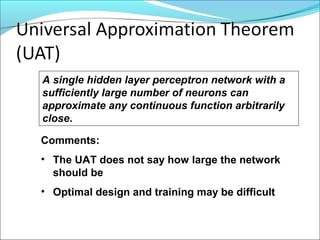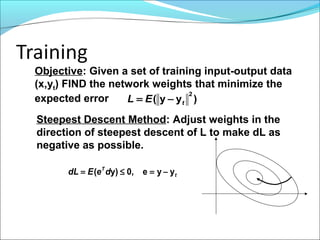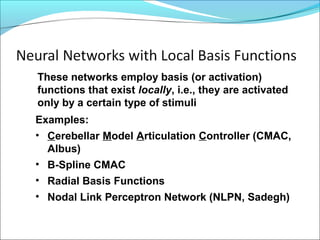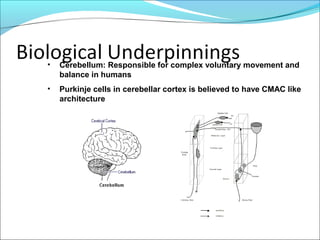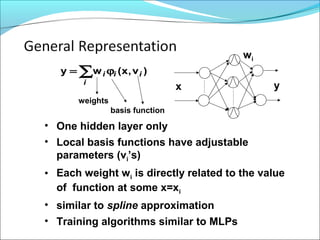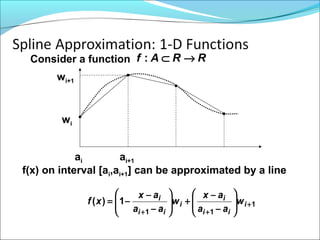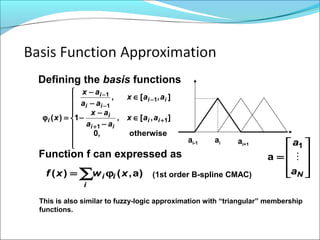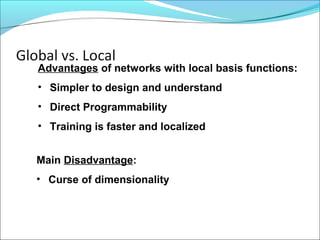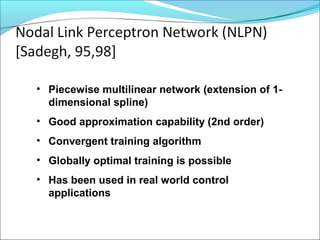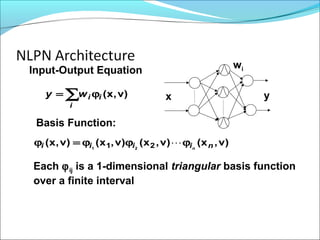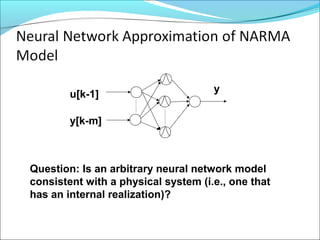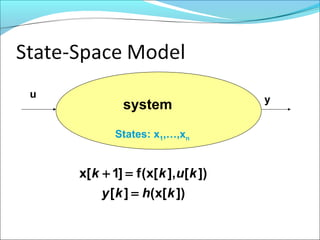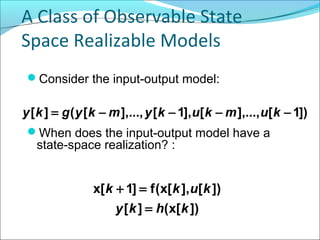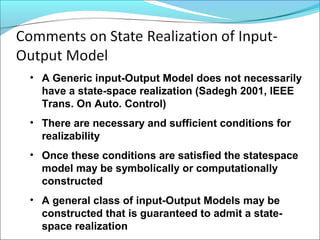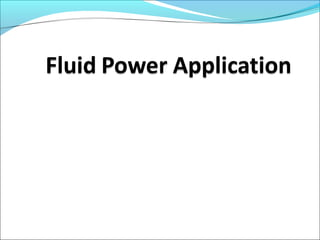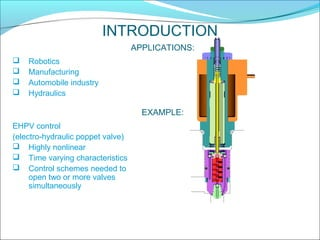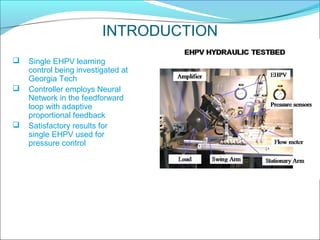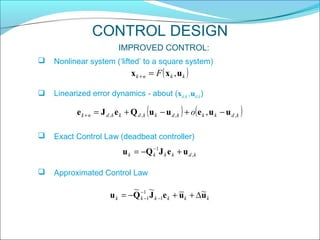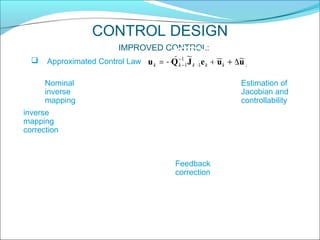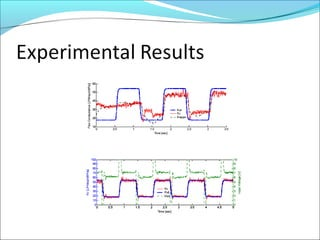1 sur 66
Publicité

### Unit1 pg math model

1. Mathematical Modeling & Analysis PG Mechanical Engineering Design Module Lecture Slides: July-December 2013 Prof. (Dr.) Gaurang V. Shah Doctor of Engineering University of Massachusetts Lowell, USA Mechanical Engineering Department, PVPIT, Bavdhan Pune
2. What is Mathematical Model? A set of mathematical equations (e.g., differential eqs.) that describes the input-output behavior of a system. What is a model used for? • Simulation • Prediction/Forecasting • Prognostics/Diagnostics • Design/Performance Evaluation • Control System Design
3. Definition of System System: An aggregation or assemblage of things so combined by man or nature to form an integral and complex whole. From engineering point of view, a system is defined as an interconnection of many components or functional units act together to perform a certain objective, e.g., automobile, machine tool, robot, aircraft, etc.
4. To every system there corresponds three sets of variables: Input variables originate outside the system and are not affected by what happens in the system Output variables are the internal variables that are used to monitor or regulate the system. They result from the interaction of the system with its environment and are influenced by the input variables System u y
5. Dynamic Systems A system is said to be dynamic if its current output may depend on the past history as well as the present values of the input variables. Mathematically, Time:Input,: ]0),([)( tu tuty ≤τ≤τϕ= Example: A moving mass M y u Model: Force=Mass x Acceleration uyM =
6. Velocity-Force: ∫ ττ+== t du M ytytv 0 )( 1 )0()()(  Therefore, this is a dynamic system. If the drag force (bdx/dt) is included, then uybyM =+  2nd order ordinary differential equation (ODE) Position-Force: dsdu M ytyty t s ∫∫ ττ++= 0 0 )( 1 )0()0()( 
7. Mathematical model of a real world system is derived using a combination of physical laws (1st principles) and/or experimental means • Physical laws are used to determine the model structure (linear or nonlinear) and order. • The parameters of the model are often estimated and/or validated experimentally. • Mathematical model of a dynamic system can often be expressed as a system of differential (difference in the case of discrete-time systems) equations
8. Input-output differential or difference equation State equations (system of 1st order eqs.) Transfer function Nonlinear Linear Linear Time Invariant System Type Model Type
9. • A nonlinear model is often linearized about a certain operating point • Model reduction (or approximation) may be needed to get a lumped-parameter (finite dimensional) model • Numerical values of the model parameters are often approximated from experimental data by curve fitting.
10. Differential Equations (Continuous-Time Systems) ubububyayayay nn n nn nn +++=++++ − − − −  1 )1( 11 )1( 1 )( )()1()()1()( 11 nkubkubnkyakyaky nn −++−+−++−=  Difference Equations (Discrete-Time Systems) Discretization Inverse Discretization
11. Consider the mass-spring-damper (may be used as accelerometer or seismograph) system shown below: Free-Body-Diagram M fs fd fs fd x fs(y): position dependent spring force, y=x-u fd(y): velocity dependent spring force Newton’s 2nd law ( ) )()( yfyfuyMxM sd −−=+=  Linearizaed model: uMkyybyM  =++ M ux
12. Delay z -1 u y Consider the digital system shown below: Input-Output Eq.: )1()1()( −+−= kukyky Equivalent to an integrator: ∑ − = = 1 0 )()( k j juky
13. Transfer Function is the algebraic input-output relationship of a linear time-invariant system in the s (or z) domain GU Y dt d s kbsms ms sU sY sGukyybym ≡ ++ ==⇔=++ , )( )( )( 2 2  Example: Accelerometer System Example: Digital Integrator ≡ − ==⇔−+−= − − z z z zu zY Gkukyky , 1)( )( )1()1()( 1 1 Forward shift
14. • Transfer function is a property of the system independent from input-output signal • It is an algebraic representation of differential equations • Systems from different disciplines (e.g., mechanical and electrical) may have the same transfer function • Chemical system of reacting species can be represented by TF • Electromagnetic wave like LASER rep by TF(?).
15. • Most systems in mechatronics are of the mixed type, e.g., electromechanical, hydromechanical, etc • Each subsystem within a mixed system can be modeled as single discipline system first • Power transformation among various subsystems are used to integrate them into the entire system • Overall mathematical model may be assembled into a system of equations, or a transfer function
16. voltageemf-backe,e dt di LiRu bb a aaa =++= Mechanical Subsystem BωωJTmotor +=  u ia dc Ra La J ω BInput: voltage u Output: Angular velocity ω Elecrical Subsystem (loop method):
17. u ia dc Ra La ω Torque-Current: Voltage-Speed: atmotor iKT = Combing previous equations results in the following mathematical model: B Power Transformation: ωKe bb =     =+ =ω++ 0at baa a a iK-BωωJ uKiR dt di L  where Kt: torque constant, Kb: velocity constant For an ideal motor bt KK =
18. Taking Laplace transform of the system’s differential equations with zero initial conditions gives: Eliminating Ia yields the input-output transfer function ( ) btaaa 2 a t KKBRBLJRJsL K U(s) Ω(s) ++++ = u ia Kt Ra La ω B( ) ( )   =Ω+ =Ω++ 0)( )()()( sIK-(s)BJs sUsKsIRsL at baaa
19. Assuming small inductance, La ≈0 ( ) ( )abt at RKKBJs RK U(s) Ω(s) ++ = which is equivalent to ω at RuK Babt RKK • The D.C. motor provides an input torque and an additional damping effect known as back-emf damping
20. • A brushless PMSM has a wound stator, a PM rotor assembly and a position sensor. • The combination of inner PM rotor and outer windings offers the advantages of – low rotor inertia – efficient heat dissipation, and – reduction of the motor size.
21. a q b c d θe θe=p θ+ θ0 Electrical angle Number of poles/2 offset θ
22. q me dmq q dqmd d v LL K ipi L R dt di v L ipi L R dt di 1 1 + ω −ω− − = +ω+ − = Where p=number of poles/2, Ke=back emf constant
23. • Parametric Identification: The input-output model coefficients are estimated to “fit” the input-output data. • Frequency-Domain (non-parametric): The Bode diagram [G(jw) vs. w in log-log scale] is estimated directly form the input-output data. The input can either be a sweeping sinusoidal or random signal. Experimental determination of system model. There are two methods of system identification:
24. u ia Kt Ra La ω B ( ) ( ) 1+ = ++ = Ts k RKKBJs RK U(s) Ω(s) abt at Transfer Function, La=0: 0 0.1 0.2 0.3 0.4 0.5 0 2 4 6 8 10 12 Time (secs) Amplitude ku T u t k=10, T=0.1
25. Graphical method is • difficult to optimize with noisy data and multiple data sets • only applicable to low order systems • difficult to automate
26. Given a linear system with uniformly sampled input output data, (u(k),y(k)), then noisenkubkubnkyakyaky nn +−++−+−++−= )()1()()1()( 11  Least squares curve-fitting technique may be used to estimate the coefficients of the above model called ARMA (Auto Regressive Moving Average) model.
27. Input: Random or deterministic Random Noise u Output n plant Noise model • persistently exciting with as much power as possible; • uncorrelated with the disturbance • as long as possible y
28. • Analytical • Experimental – Time response analysis (e.g., step, impulse) – Parametric * ARX, ARMAX * Box-Jenkins * State-Space – Nonparametric or Frequency based * Spectral Analysis (SPA) * Emperical Transfer Function Analysis (ETFE)
29. Bode Diagram of 10 -1 10 0 10 1 10 2 -10 0 10 20 Frequency (rad/sec) GaindB 10 -1 10 0 10 1 10 2 -30 -60 -90 0 Frequency (rad/sec) Phasedeg 1/T 20log( )k 1 )( + = Ts k sG
30. Method I (Sweeping Sinusoidal): systemAi Ao f t>>0 Magnitude Phase db =       = A Ai 0 , φ Method II (Random Input): system Transfer function is determined by analyzing the spectrum of the input and output
31. • Pointwise Estimation: )( )( )( ω ω =ω U Y jG This often results in a very nonsmooth frequency response because of data truncation and noise. • Spectral estimation: uses “smoothed” sample estimators based on input-output covariance and crosscovariance. The smoothing process reduces variability at the expense of adding bias to the estimate )(ˆ )(ˆ )(ˆ ωΦ ωΦ =ω u yuj eG
32. 0.1 1 10 100 1 10 375 50 25 0 25 Frequency (Hz) Magnitude(dB) 0.1 1 10 100 1 10 3180 90 0 90 180 Frequency (Hz) Phase(Deg) high order low order
33. Neural Network Approach
34. Prof. (Dr.) Gaurang Shah PVPIT, Pune July-December 2013 Lecture Notes: Mathematical Modeling Analysis PG ME (Design)
35. Introduction Real world nonlinear systems often difficult to characterize by first principle modeling First principle models are often suitable for control design Modeling often accomplished with input-output maps of experimental data from the system Neural networks provide a powerful tool for data- driven modeling of nonlinear systems
36. ])1[],...,[],1[],...,[(][ −−−−= kumkukymkygky g z -1 z -1 z -1 z -1 z -1 z -1 u y
37. • Artificial Neural Networks (ANN) are massively parallel computational machines (program or hardware) patterned after biological neural nets. • ANN’s are used in a wide array of applications requiring reasoning/information processing including –pattern recognition/classification –monitoring/diagnostics –system identification & control –forecasting –optimization
38. • Learning from examples rather than “hard” programming • Ability to deal with unknown or uncertain situations • Parallel architecture; fast processing if implemented in hardware • Adaptability • Fault tolerance and redundancy
39. • Hard to design • Unpredictable behavior • Slow Training • “Curse” of dimensionality
40. • A neuron is a building block of biological networks • A single cell neuron consists of the cell body (soma), dendrites, and axon. • The dendrites receive signals from axons of other neurons. • The pathway between neurons is synapse with variable strength
41. Artificial Neural Networks They are used to learn a given input-output relationship from input-output data (exemplars). Most popular ANNs: Multilayer perceptron Radial basis function CMAC
42. Objective: Find a finite-dimensional representation of a function with compact domain • Classical Techniques -Polynomial, Trigonometric, Splines • Modern Techniques -Neural Nets, Fuzzy-Logic, Wavelets, etc. mn RRAf →⊂:
43. x1 x2 y • MLP is used to learn, store, and produce input output relationships Training: network are adjusted to match a set of known input-output (x,y) training data Recall: produces an output according to the learned weights
44. x y Wk,ij: Weight from node i in layer k-1 to node j in layer k ( )( )( )( )xWσWσσWσWy TTT p T p 011 −= σ: Activation function, e.g.,               + + = − − nx x e e 1 1 1 1 )( 1 xσ W0 Wp p: number of hidden layers
45. A single hidden layer perceptron network with a sufficiently large number of neurons can approximate any continuous function arbitrarily close. Comments: • The UAT does not say how large the network should be • Optimal design and training may be difficult
46. Objective: Given a set of training input-output data (x,yt) FIND the network weights that minimize the expected error )yy( 2 tEL −= Steepest Descent Method: Adjust weights in the direction of steepest descent of L to make dL as negative as possible. t T deEdL yye,0)y( −=≤=
47. Examples: • Cerebellar Model Articulation Controller (CMAC, Albus) • B-Spline CMAC • Radial Basis Functions • Nodal Link Perceptron Network (NLPN, Sadegh) These networks employ basis (or activation) functions that exist locally, i.e., they are activated only by a certain type of stimuli
48. • Cerebellum: Responsible for complex voluntary movement and balance in humans • Purkinje cells in cerebellar cortex is believed to have CMAC like architecture
49. x y )v,x(wy i i ii∑ ϕ= weights basis function • One hidden layer only • Local basis functions have adjustable parameters (vi’s) • Each weight wi is directly related to the value of function at some x=xi • similar to spline approximation • Training algorithms similar to MLPs wi
50. Consider a function RRAf →⊂: f(x) on interval [ai,ai+1] can be approximated by a line ai+1 wi ai wi+1 1 11 1)( + ++       − − +      − − −= i ii i i ii i w aa ax w aa ax xf
51. Defining the basis functions )a,()( xwxf i ii∑ ϕ=          ∈ − − − ∈ − − =ϕ + + − − − otherwise,0 ],[,1 ],[, )( 1 1 1 1 1 ii ii i ii ii i i aax aa ax aax aa ax x aiai-1 ai+1 Function f can expressed as           = Na a  1 a This is also similar to fuzzy-logic approximation with “triangular” membership functions. (1st order B-spline CMAC)
52. Advantages of networks with local basis functions: • Simpler to design and understand • Direct Programmability • Training is faster and localized Main Disadvantage: • Curse of dimensionality
53. • Piecewise multilinear network (extension of 1- dimensional spline) • Good approximation capability (2nd order) • Convergent training algorithm • Globally optimal training is possible • Has been used in real world control applications
54. x y wi )v,x(∑ ϕ= i iiwy )v,x()v,x()v,x()v,x( 21 21 niiii n ϕϕϕ=ϕ  Input-Output Equation Basis Function: Each ϕij is a 1-dimensional triangular basis function over a finite interval
55. y y[k-m] u[k-1] Question: Is an arbitrary neural network model consistent with a physical system (i.e., one that has an internal realization)?
56. ])[x(][ ])[],[x(f]1[x khky kukk = =+ u y States: x1,…,xn system
57. A Class of Observable State Space Realizable Models Consider the input-output model: When does the input-output model have a state-space realization? : ])[x(][ ])[],[x(f]1[x khky kukk = =+ ])1[],...,[],1[],...,[(][ −−−−= kumkukymkygky
58. • A Generic input-Output Model does not necessarily have a state-space realization (Sadegh 2001, IEEE Trans. On Auto. Control) • There are necessary and sufficient conditions for realizability • Once these conditions are satisfied the statespace model may be symbolically or computationally constructed • A general class of input-Output Models may be constructed that is guaranteed to admit a state- space realization
59. Prof. (Dr.) Gaurang Shah PVPIT, Pune July-December 2013 Lecture slideMathematical Modeling AnalysisPG Mechanical Engineering (Design)
60. APPLICATIONS:  Robotics  Manufacturing  Automobile industry  Hydraulics INTRODUCTION EHPV control (electro-hydraulic poppet valve)  Highly nonlinear  Time varying characteristics  Control schemes needed to open two or more valves simultaneously EXAMPLE:
61. EXAMPLE:  Single EHPV learning control being investigated at Georgia Tech  Controller employs Neural Network in the feedforward loop with adaptive proportional feedback  Satisfactory results for single EHPV used for pressure control INTRODUCTION
62. IMPROVED CONTROL:  Linearized error dynamics - about (xd,k ,ud,k) CONTROL DESIGN ( ) ( )kdkkkdkkdkkdnk o ,,,, , uueuuQeJe −+−+=+ kdkkkk , 1 ueJQu +−= −  Exact Control Law (deadbeat controller)  Nonlinear system (‘lifted’ to a square system) ( )kknk F uxx ,=+  Approximated Control Law kkkkkk uueJQu ~~~ 1 1 1 ∆++−= − − −
63. IMPROVED CONTROL: CONTROL DESIGN kkkkkk uueJQu ~~~ 1 1 1 ∆++−= − − −  Approximated Control Law Estimation of Jacobian and controllability Feedback correction Nominal inverse mapping inverse mapping correction
Publicité